# SCT-013-030 current monitor sensor

• Hello,

Do you now if someone already made a sketch for the SCT-013-030 sensors ?
I'm trying to find something on google, but I haven't found nothing for now.

Thanks !

• @chatainsim said:

SCT-013-030

I create custom energy monitor and use mysesnors to send data to server.
http://forum.mysensors.org/topic/703/custom-power-meter/4
My final sketch

``````#include <SPI.h>
#include <MySensor.h>
#include "EmonLib.h"             // Include Emon Library
EnergyMonitor emon1;             // Create an instance
EnergyMonitor emon2;             // Create an instance
EnergyMonitor emon3;             // Create an instance
#define CHILD_ID 0
#define CHILD_ID2 1
#define CHILD_ID3 2
MySensor gw;
unsigned long lastSend;
unsigned long SEND_FREQUENCY = 10000; // Minimum time between send (in milliseconds). We don't wnat to spam the gateway.
unsigned long lastSend2;
unsigned long SEND_FREQUENCY2 = 300000; // Minimum time between send (in milliseconds). We don't wnat to spam the gateway.

MyMessage realPowerMsg(CHILD_ID,V_VAR1);
MyMessage apparentPowerMsg(CHILD_ID,V_VAR2);
MyMessage powerFActorMsg(CHILD_ID,V_VAR3);
MyMessage supplyVoltageMsg(CHILD_ID,V_VAR4);
MyMessage IrmsMsg(CHILD_ID,V_VAR5);

MyMessage realPowerMsg2(CHILD_ID2,V_VAR1);
MyMessage apparentPowerMsg2(CHILD_ID2,V_VAR2);
MyMessage powerFActorMsg2(CHILD_ID2,V_VAR3);
MyMessage supplyVoltageMsg2(CHILD_ID2,V_VAR4);
MyMessage IrmsMsg2(CHILD_ID2,V_VAR5);

MyMessage realPowerMsg3(CHILD_ID3,V_VAR1);
MyMessage apparentPowerMsg3(CHILD_ID3,V_VAR2);
MyMessage powerFActorMsg3(CHILD_ID3,V_VAR3);
MyMessage supplyVoltageMsg3(CHILD_ID3,V_VAR4);
MyMessage IrmsMsg3(CHILD_ID3,V_VAR5);

void setup()
{
gw.begin(NULL, 4, false, 0);
Serial.begin(115200); // opens serial port, sets data rate to 9600 bps
// Send the sketch version information to the gateway and Controller
gw.sendSketchInfo("Energy Meter x 3v2", "1.2");
// Register this device as power sensor
gw.present(CHILD_ID, S_POWER);
gw.present(CHILD_ID2, S_POWER);
gw.present(CHILD_ID3, S_POWER);

emon1.voltage(A3, 116.26, 1.7);  // Voltage: input pin, calibration, phase_shift
emon1.current(A0, 29.0);       // Current: input pin, calibration.
emon2.voltage(A4, 116.66, 1.7);  // Voltage: input pin, calibration, phase_shift
emon2.current(A1, 29.0);       // Current: input pin, calibration.
emon3.voltage(A5, 115.91, 1.7);  // Voltage: input pin, calibration, phase_shift
emon3.current(A2, 29.0);       // Current: input pin, calibration.
}

void loop()
{
gw.process();
unsigned long now = millis();
emon1.calcVI(20,2000);         // Calculate all. No.of half wavelengths (crossings), time-out
//emon1.serialprint();           // Print out all variables (realpower, apparent power, Vrms, Irms, power factor)
emon2.calcVI(20,2000);         // Calculate all. No.of half wavelengths (crossings), time-out
//emon2.serialprint();           // Print out all variables (realpower, apparent power, Vrms, Irms, power factor)
emon3.calcVI(20,2000);         // Calculate all. No.of half wavelengths (crossings), time-out
//emon3.serialprint();           // Print out all variables (realpower, apparent power, Vrms, Irms, power factor)

bool sendTime = now - lastSend > SEND_FREQUENCY;
if (sendTime) {

float realPower       = emon1.realPower;        //extract Real Power into variable
float realPower2       = emon2.realPower;        //extract Real Power into variable
float realPower3       = emon3.realPower;        //extract Real Power into variable

if(realPower > 0 && realPower < 10000){
gw.send(realPowerMsg.set(realPower,1));
}
if(realPower2 > 0 && realPower2 < 10000){
gw.send(realPowerMsg2.set(realPower2,1));
}
if(realPower3 > 0 && realPower3 < 10000){
gw.send(realPowerMsg3.set(realPower3,1));
}
lastSend = now;
}

bool sendTime2 = now - lastSend2 > SEND_FREQUENCY2;
if(sendTime2){

float apparentPower   = emon1.apparentPower;    //extract Apparent Power into variable
float powerFactor     = emon1.powerFactor;      //extract Power Factor into Variable
float supplyVoltage   = emon1.Vrms;             //extract Vrms into Variable
float Irms            = emon1.Irms;             //extract Irms into Variable

float apparentPower2   = emon2.apparentPower;    //extract Apparent Power into variable
float powerFactor2     = emon2.powerFactor;      //extract Power Factor into Variable
float supplyVoltage2   = emon2.Vrms;             //extract Vrms into Variable
float Irms2            = emon2.Irms;             //extract Irms into Variable

float apparentPower3   = emon3.apparentPower;    //extract Apparent Power into variable
float powerFactor3     = emon3.powerFactor;      //extract Power Factor into Variable
float supplyVoltage3   = emon3.Vrms;             //extract Vrms into Variable
float Irms3            = emon3.Irms;             //extract Irms into Variable

if(apparentPower > 0 && apparentPower < 10000){
gw.send(apparentPowerMsg.set(apparentPower,1));
}
if(powerFactor > 0 && powerFactor < 10000){
gw.send(powerFActorMsg.set(powerFactor,1));
}
if(supplyVoltage > 0 && supplyVoltage < 1000){
gw.send(supplyVoltageMsg.set(supplyVoltage,1));
}
if(Irms > 0 && Irms < 1000){
gw.send(IrmsMsg.set(Irms,1));
}

if(apparentPower2 > 0 && apparentPower2 < 10000){
gw.send(apparentPowerMsg2.set(apparentPower2,1));
}
if(powerFactor2 > 0 && powerFactor2 < 10000){
gw.send(powerFActorMsg2.set(powerFactor2,1));
}
if(supplyVoltage2 > 0 && supplyVoltage2 < 1000){
gw.send(supplyVoltageMsg2.set(supplyVoltage2,1));
}
if(Irms2 > 0 && Irms2 < 1000){
gw.send(IrmsMsg2.set(Irms2,1));
}

if(apparentPower3 > 0 && apparentPower3 < 10000){
gw.send(apparentPowerMsg3.set(apparentPower3,1));
}
if(powerFactor3 > 0 && powerFactor3 < 10000){
gw.send(powerFActorMsg3.set(powerFactor3,1));
}
if(supplyVoltage3 > 0 && supplyVoltage3 < 1000){
gw.send(supplyVoltageMsg3.set(supplyVoltage3,1));
}
if(Irms3 > 0 && Irms3 < 1000){
gw.send(IrmsMsg3.set(Irms3,1));
}
lastSend2 = now;
}
}``````

• Thank you for the code.
Can you tell me how to wire the sensor ?
I have only two wire connected to the jack.

Thanks !

• This post is deleted!

• this code is for three, first one is wired on A3 / A0, second A4/A1 and third on A5/A2

• Yes, I've understand this but how is it possible to wire on A3 and A0 with only two wires ? Where is connected the VCC ?
I've used this : http://openenergymonitor.org/emon/buildingblocks/how-to-build-an-arduino-energy-monitor-measuring-current-only

• @maglo18 said:

A3 is from transformer and A0 is from CT sensor

I have 3 x voltage input and 3 x ct input.

• Ahhh ! Ok.
That why there is A3 and A0 in use !

I'll digg into the voltage input to know how it's working.
Thank you.

• I've change the code to only use A0 for CT sensor.
It's not plug in the cable, it's for testing.
Here is the output in arduino serial console :

``````sensor started, id 2
send: 2-2-0-0 s=255,c=0,t=17,pt=0,l=5,st=ok:1.4.1
send: 2-2-0-0 s=255,c=3,t=6,pt=1,l=1,st=ok:0
send: 2-2-0-0 s=255,c=3,t=11,pt=0,l=12,st=ok:Energy Meter
send: 2-2-0-0 s=255,c=3,t=12,pt=0,l=3,st=ok:2.0
send: 2-2-0-0 s=0,c=0,t=13,pt=0,l=5,st=ok:1.4.1
0.00 0.00 0.00 69.60 nan
0.00 0.00 0.00 62.07 nan
0.00 0.00 0.00 55.65 nan
0.00 0.00 0.00 48.57 nan
0.00 0.00 0.00 40.78 nan
0.00 0.00 0.00 35.21 nan
0.00 0.00 0.00 30.80 nan
0.00 0.00 0.00 24.84 nan
0.00 0.00 0.00 19.95 nan
0.00 0.00 0.00 16.46 nan
send: 2-2-0-0 s=0,c=1,t=24,pt=7,l=5,st=ok:0.0
send: 2-2-0-0 s=0,c=1,t=25,pt=7,l=5,st=ok:0.0
send: 2-2-0-0 s=0,c=1,t=26,pt=7,l=5,st=ok:NAN
send: 2-2-0-0 s=0,c=1,t=27,pt=7,l=5,st=fail:0.0
send: 2-2-0-0 s=0,c=1,t=28,pt=7,l=5,st=fail:16.5
0.00 0.00 0.00 13.53 nan
0.00 0.00 0.00 11.34 nan
0.00 0.00 0.00 9.73 nan
0.00 0.00 0.00 8.11 nan
0.00 0.00 0.00 6.84 nan
0.00 0.00 0.00 5.72 nan
0.00 0.00 0.00 4.71 nan
0.00 0.00 0.00 3.90 nan
0.00 0.00 0.00 3.18 nan
0.00 0.00 0.00 2.61 nan
send: 2-2-0-0 s=0,c=1,t=24,pt=7,l=5,st=ok:0.0
send: 2-2-0-0 s=0,c=1,t=25,pt=7,l=5,st=ok:0.0
send: 2-2-0-0 s=0,c=1,t=26,pt=7,l=5,st=ok:NAN
send: 2-2-0-0 s=0,c=1,t=27,pt=7,l=5,st=fail:0.0
send: 2-2-0-0 s=0,c=1,t=28,pt=7,l=5,st=fail:2.6
0.00 0.00 0.00 2.22 nan
0.00 0.00 0.00 1.97 nan
0.00 0.00 0.00 1.76 nan
0.00 0.00 0.00 1.62 nan
0.00 0.00 0.00 1.51 nan
0.00 0.00 0.00 1.40 nan
0.00 0.00 0.00 1.26 nan
0.00 0.00 0.00 1.13 nan
0.00 0.00 0.00 0.99 nan
0.00 0.00 0.00 0.85 nan
send: 2-2-0-0 s=0,c=1,t=24,pt=7,l=5,st=ok:0.0
send: 2-2-0-0 s=0,c=1,t=25,pt=7,l=5,st=ok:0.0
send: 2-2-0-0 s=0,c=1,t=26,pt=7,l=5,st=ok:NAN
send: 2-2-0-0 s=0,c=1,t=27,pt=7,l=5,st=fail:0.0
send: 2-2-0-0 s=0,c=1,t=28,pt=7,l=5,st=fail:0.8
0.00 0.00 0.00 0.74 nan
0.00 0.00 0.00 0.65 nan
0.00 0.00 0.00 0.57 nan
0.00 0.00 0.00 0.50 nan
0.00 0.00 0.00 0.40 nan
0.00 0.00 0.00 0.33 nan
0.00 0.00 0.00 0.29 nan
0.00 0.00 0.00 0.25 nan
0.00 0.00 0.00 0.23 nan
0.00 0.00 0.00 0.20 nan
send: 2-2-0-0 s=0,c=1,t=24,pt=7,l=5,st=ok:0.0
send: 2-2-0-0 s=0,c=1,t=25,pt=7,l=5,st=ok:0.0
send: 2-2-0-0 s=0,c=1,t=26,pt=7,l=5,st=ok:NAN
send: 2-2-0-0 s=0,c=1,t=27,pt=7,l=5,st=fail:0.0
send: 2-2-0-0 s=0,c=1,t=28,pt=7,l=5,st=fail:0.2
0.00 0.00 0.00 0.19 nan
0.00 0.00 0.00 0.16 nan
0.00 0.00 0.00 0.13 nan
0.00 0.00 0.00 0.14 nan
0.00 0.00 0.00 0.14 nan
0.00 0.00 0.00 0.11 nan
0.00 0.00 0.00 0.11 nan
0.00 0.00 0.00 0.09 nan
``````

There are some fail, is it normal ?
Thanks

• I starting to understand.
The SCT-013-030 is used only to get Ampere, nothing more ? Is it correct ?
If I want the Watt I need more sensors connected to the arduino, right ?

• @chatainsim, if you know the Voltage, just multiply the voltage by the amperage and you will have the wattage...

http://en.wikipedia.org/wiki/Ohm's_law

• In Emonlib you have example to measure watt etc. without measure voltage.
https://github.com/openenergymonitor/EmonLib

• @rvendrame Thanks, I will try this.
@maglo18 I will try to use some example.
First I need to check if my sketch and my assemble works at least.

Thanks for your help. I think I will need more from you guys !

• Hello guys,

I've working on my sensor.
It's seems working, but I have some trouble with Watt or Kwh.

Here is the sketch:

``````// EmonLibrary examples openenergymonitor.org, Licence GNU GPL V3  ***/
#include <SPI.h>
#include <MySensor.h>
#include "EmonLib.h"             // Include Emon Library
EnergyMonitor emon1;             // Create an instance
#define CHILD_ID 0
MySensor gw;
unsigned long lastSend;
unsigned long SEND_FREQUENCY = 20000; // Minimum time between send (in milliseconds). We don't wnat to spam the gateway.

MyMessage IrmsMsg(CHILD_ID,V_WATT);
void setup()
{
gw.begin();

// Send the sketch version information to the gateway and Controller
gw.sendSketchInfo("Energy Meter", "1.0");

// Register this device as power sensor
gw.present(CHILD_ID, S_POWER);
emon1.current(A0, 29.0);       // Current: input pin, calibration.
}

void loop()
{
gw.process();
unsigned long now = millis();
double Irms = emon1.calcIrms(1480);
//emon1.calcVI(20,2000);         // Calculate all. No.of half wavelengths (crossings), time-out
//emon1.serialprint();           // Print out all variables (realpower, apparent power, Vrms, Irms, power factor)
bool sendTime = now - lastSend > SEND_FREQUENCY;
if (sendTime) {
//float Irms = emon1.Irms;             //extract Irms into Variable
gw.send(IrmsMsg.set(Irms*232.0,1));
Serial.println(Irms*232.0);
lastSend = now;
}
}
``````

I've got around 390 for the Irms*232, do you thinks it's a correct value ?
Can I calculated the current kwh with the ampere ?

Thank you.

• Hello,

I've made some change in the code :

``````// EmonLibrary examples openenergymonitor.org, Licence GNU GPL V3  ***/
#include <SPI.h>
#include <MySensor.h>
#include "EmonLib.h"             // Include Emon Library
EnergyMonitor emon1;             // Create an instance
#define CHILD_ID_WATT 0
#define CHILD_ID_KWH 1
MySensor gw;
unsigned long lastSend;
unsigned long SEND_FREQUENCY = 20000; // Minimum time between send (in milliseconds). We don't wnat to spam the gateway.

MyMessage IrmsMsg(CHILD_ID_WATT,V_WATT);
MyMessage kWhMsg(CHILD_ID_KWH,V_KWH);
void setup()
{
gw.begin();
gw.sendSketchInfo("Energy Meter", "1.0");  // Send the sketch version information to the gateway and Controller
gw.present(CHILD_ID_WATT, S_POWER);  // Register this device as power sensor
gw.present(CHILD_ID_KWH, S_POWER);
emon1.current(A0, 29.0);       // Current: input pin, calibration.
}

void loop()
{
gw.process();
unsigned long now = millis();
//emon1.serialprint();           // Print out all variables (realpower, apparent power, Vrms, Irms, power factor)
double Irms = emon1.calcIrms(1480);
bool sendTime = now - lastSend > SEND_FREQUENCY;
if (sendTime) {
gw.send(IrmsMsg.set((Irms*232.0)*1000, 1));
//    Serial.print("Watt: ");
//    Serial.println(Irms*232.0);
gw.send(kWhMsg.set((Irms*232.0)/1000, 1));
//    Serial.print("kWH: ");
//    Serial.println((Irms*232.0)/1000);
lastSend = now;
}
}
``````

But in Domoticz, I've two line for this but in kWh and not on in kWh and other in Watt :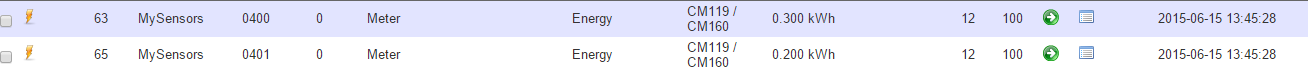Do you know why ?

Regards,

• @chatainsim Domoticz does not have a different device for power (Watt) and energy(kWh) but combines them in a single device. What you need to do is send both the power and energy to the same child.

• Thanks, but I've already test this and only the kWh is updated in domoticz, not watt.

``````// EmonLibrary examples openenergymonitor.org, Licence GNU GPL V3  ***/
#include <SPI.h>
#include <MySensor.h>
#include "EmonLib.h"             // Include Emon Library
EnergyMonitor emon1;             // Create an instance
#define CHILD_ID 0
MySensor gw;
unsigned long lastSend;
unsigned long SEND_FREQUENCY = 20000; // Minimum time between send (in milliseconds). We don't wnat to spam the gateway.

MyMessage IrmsMsg(CHILD_ID,V_WATT);
MyMessage kWhMsg(CHILD_ID,V_KWH);
void setup()
{
gw.begin();
gw.sendSketchInfo("Energy Meter", "1.0");  // Send the sketch version information to the gateway and Controller
gw.present(CHILD_ID, S_POWER);  // Register this device as power sensor
emon1.current(A0, 29.0);       // Current: input pin, calibration.
}

void loop()
{
gw.process();
unsigned long now = millis();
//emon1.serialprint();           // Print out all variables (realpower, apparent power, Vrms, Irms, power factor)
double Irms = emon1.calcIrms(1480);
bool sendTime = now - lastSend > SEND_FREQUENCY;
if (sendTime) {
gw.send(IrmsMsg.set((Irms*232.0)*1000, 1));
//    Serial.print("Watt: ");
//    Serial.println(Irms*232.0);
gw.send(kWhMsg.set((Irms*232.0)/1000, 1));
//    Serial.print("kWH: ");
//    Serial.println((Irms*232.0)/1000);
lastSend = now;
}
}
``````

Can you help me with this ?

Thanks

• @chatainsim I am pretty sure it is a combined device which shows Watt only when added ...: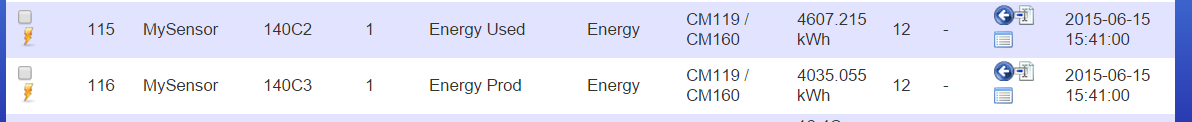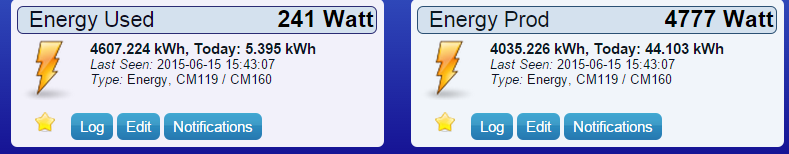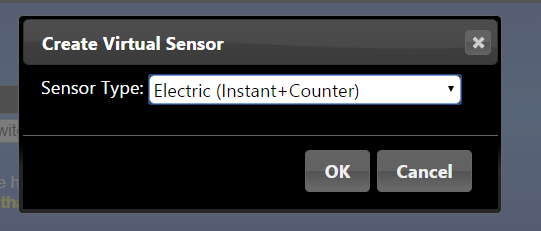Although I am not sure if the Domoticz MySensors plug-in handles it right (I'm still using my own script).

• Ok, thanks.
How do you get your watt info ? With teleinfo ?

• @chatainsim
I send the V_Watt messages from the Power meter to Domoticz.

• @AWI

MyMessage IrmsMsg(CHILD_ID,V_WATT);
MyMessage kWhMsg(CHILD_ID,V_KWH);

I also send both data but it's seems that Domoticz is ingnoring it !
I don't know why.

• @chatainsim Sounds you like you need to consult the Domoticz forum. The Domoticz code shows that is interpreted.

``````case V_WATT:
{
_tMySensorSensor *pSensorKwh = FindSensor(pSensor->nodeID, V_KWH);
if (pSensorKwh) {
SendKwhMeter(pSensorKwh->nodeID, pSensorKwh->childID, pSensorKwh->batValue, pSensor->floatValue / 1000.0f, pSensorKwh->floatValue, "Meter");
}
else {
_tUsageMeter umeter;
umeter.id1 = 0;
umeter.id2 = 0;
umeter.id3 = 0;
umeter.id4 = pSensor->nodeID;
umeter.dunit = pSensor->childID;
umeter.fusage = pSensor->floatValue/1000.0f;
sDecodeRXMessage(this, (const unsigned char *)&umeter);
}
}
break;
case V_KWH:
{
_tMySensorSensor *pSensorWatt = FindSensor(pSensor->nodeID, V_WATT);
if (pSensorWatt) {
SendKwhMeter(pSensor->nodeID, pSensor->childID, pSensor->batValue, pSensorWatt->floatValue / 1000.0f, pSensor->floatValue, "Meter");
}
else {
SendKwhMeter(pSensor->nodeID, pSensor->childID, pSensor->batValue, 0, pSensor->floatValue, "Meter");
}
}
break;`````````

• Does anyone have some OpenHab items and rules for @chatainsim sketch posted above? I have a SCT-013-000 can anyone help me use it in my project?
Thanks!

• Hello, anybody can help me here?
What I want to do is to get the instant watt or kW read from my SCT013000 and to get also the kWh from a certain date, let's say fro first of this month!
Any idea will be kindly appreciated!
I'm using openhanb to display info and I'll need some rules for this!
Thanks all!

• Nobody here?

• I have got this to work on my Cdn system 120/240 vac using A0 and A1 pins. The code needed to be changed to address our voltage and another clamp added., but I do have a few questions;

1. Is there a way I can record or view amps consumed in the vera dashboard?

2. I used the sketch above displayed by @chatainsim and in it there are two lines of code with values 29.0 and second 1480 both in green and in brackets. For the life of me I can not find an explanation of these or how the values were arrived at. Can anyone help with explanation?

``````  gw.present(CHILD_ID, S_POWER);
emon1.current(A0, 29.0);       // Current: input pin, calibration.
}

void loop()
{
gw.process();
unsigned long now = millis();
double Irms = emon1.calcIrms(1480);
``````
3. Is there a method to show current in phase. I tried presenting CHILD_ID, S_MULTIMETER and MyMessage IrmsMsg(CHILD_ID,V_CURRENT); but got nowhere.

4. There is/was much running up and down stairs from electrical panel to computer and back is OTA IDE what I think it might be-- firmware or sketches can be updated over the air from my computer>vera>gw>node while not needing to access the node? If so there is much learning for me to do.

5. Using arduino 1.6.5, Lib 1.4.1, vera 3/veralite, ui5, Ethernet g/w , win 8.1, pro mini clone

• Hello, last week i buy SCT013-30 (ebay.fr) and follow this topic for have powermeter with mysensors. I need only energy (kWh) and power (W) same my OWL sensors (use with domoticz controler)
I modifie the sketch from mysensors/pulse powermeter (http://www.mysensors.org/build/pulse_power)

``````// EmonLibrary examples openenergymonitor.org, Licence GNU GPL V3  ***/
#include <SPI.h>
#include <MySensor.h>
#include "EmonLib.h"             // Include Emon Library
EnergyMonitor emon1;             // Create an instance
#define CHILD_ID 0
#define PIN_ANALOG_I A2
MySensor gw;
unsigned long lastSend;
unsigned long lastSend2;
unsigned long SEND_FREQUENCY = 90000; // Minimum time between send (in milliseconds).
unsigned long SEND_FREQUENCY2 = SEND_FREQUENCY / 25;
int index = 0;
double Irms=0;
double power;
double nrj=0, old_nrj;
MyMessage IrmsMsg(CHILD_ID,V_WATT);
MyMessage kWhMsg(CHILD_ID,V_KWH);
MyMessage pcMsg(CHILD_ID,V_VAR1);

void incomingMessage(const MyMessage &message)
{
if (message.type==V_VAR1)
{
nrj = old_nrj = message.getLong();
Serial.print("Received last nrj count from gw:");
Serial.println(nrj);
}
}

void setup()
{
gw.begin(incomingMessage);
gw.sendSketchInfo("Energy Meter", "1.0");  // Send the sketch version information to the gateway and Controller
gw.present(CHILD_ID, S_POWER);   // Register this device as power sensor
gw.request(CHILD_ID, V_VAR1);
emon1.current(PIN_ANALOG_I, 30.0);       // Current: input pin, calibration.
}

void loop()
{
gw.process();
unsigned long now = millis();
unsigned long now2 = millis();
bool sendTime2 = now2 - lastSend2 > SEND_FREQUENCY2;
if (sendTime2) //calcul Irms moy
{
if (index==0) Irms=emon1.calcIrms(1480);
else {
index++;
Irms = (index*Irms+emon1.calcIrms(1480))/(index+1);
}
lastSend2 = now2;
}
bool sendTime = now - lastSend > SEND_FREQUENCY;
{
power = Irms*232.0;
gw.send(IrmsMsg.set(power,1));
Serial.println(Irms*232.0);
nrj += (power*SEND_FREQUENCY/1000)/3.6E6;
gw.send(kWhMsg.set(nrj,3));
lastSend = now;
index = 0;
old_nrj=nrj;
}
{
gw.request(CHILD_ID, V_VAR1);
lastSend=now;
}
}
``````

it seems that it works for now (but not optimal).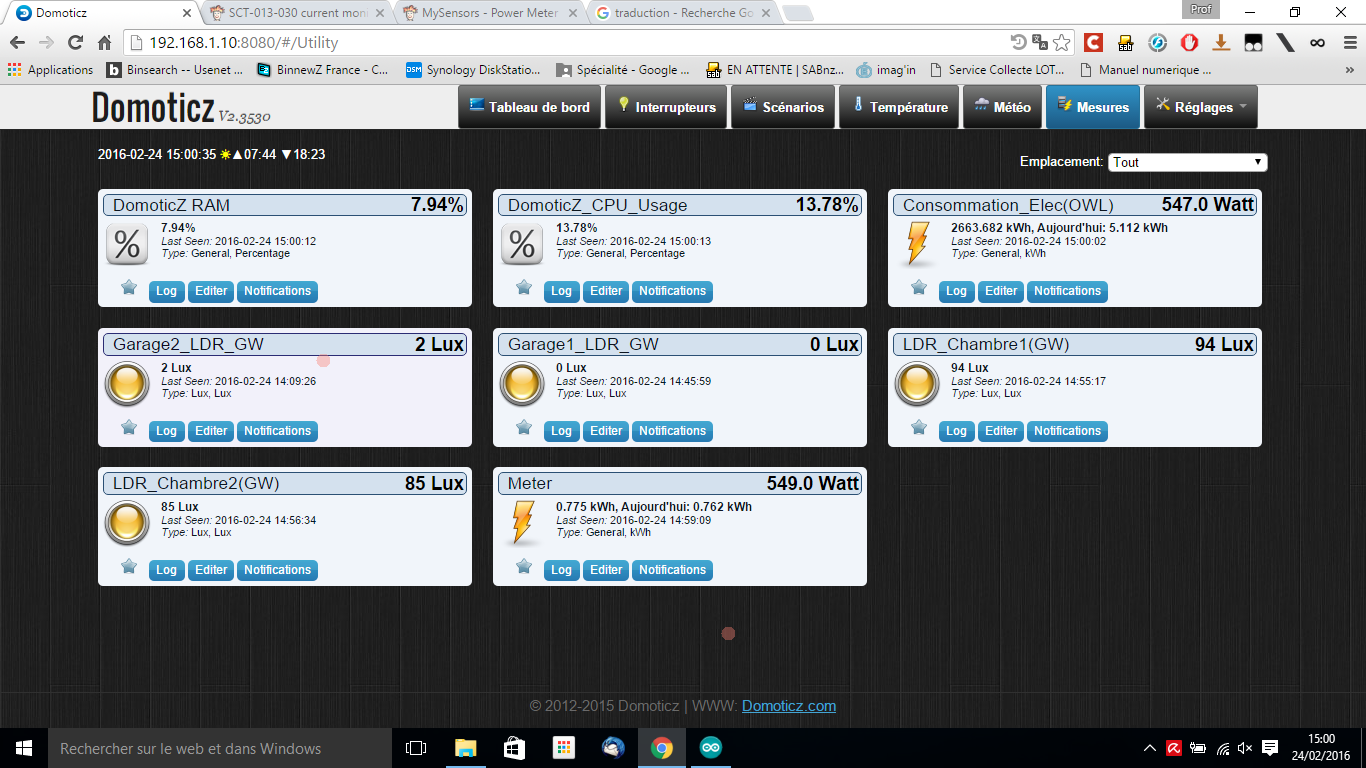Note 1) my cosphy=1 because i use only ampere clamp, i cant measure the phase between curent i and tension v.
2) my calibration constant (used in emonlib) is 30
3) Sorry for my english- Je suis français.

• Hello,
The cos phi applies only to the three-phase, single phase not

Je suis français aussi...

• Hello, if i remember physics course in university Power P=u.i and energy E=integrale(Pdt)=integrale(u.i.dt)=UeffxIeffxcos(phi)
but for particular citizen same me, energy provider non consider cos(phi)

So, i correct my last sketch for request last nrj value to the gw.

``````// EmonLibrary examples openenergymonitor.org, Licence GNU GPL V3  ***/
#include <SPI.h>
#include <MySensor.h>
#include "EmonLib.h"             // Include Emon Library
EnergyMonitor emon1;             // Create an instance
#define CHILD_ID 0
#define PIN_ANALOG_I A2
MySensor gw;
unsigned long lastSend;
unsigned long lastSend2;
unsigned long SEND_FREQUENCY = 20000; // Minimum time between send (in milliseconds). We don't wnat to spam the gateway.
unsigned long SEND_FREQUENCY2 = SEND_FREQUENCY / 25;
int index = 0;
double Irms=0;
double power;
boolean onyva=true;
float nrj=0, old_nrj;
MyMessage IrmsMsg(CHILD_ID,V_WATT);
MyMessage kWhMsg(CHILD_ID,V_KWH);
MyMessage pcMsg(CHILD_ID,V_VAR1);

void incomingMessage(const MyMessage &message)
{
if (message.type==V_VAR1)
{
nrj = old_nrj = message.getFloat();
Serial.print("Received last nrj count from gw:");
Serial.println(nrj);
}
}

void setup()
{
gw.begin(incomingMessage);
gw.sendSketchInfo("Energy Meter", "1.0");  // Send the sketch version information to the gateway and Controller
gw.present(CHILD_ID, S_POWER);   // Register this device as power sensor
gw.request(CHILD_ID, V_VAR1);
emon1.current(PIN_ANALOG_I, 30.0);       // Current: input pin, calibration.
}

void loop()
{
if (onyva) gw.process();
onyva = false;
unsigned long now = millis();
//unsigned long now2 = millis();
bool sendTime2 = now - lastSend2 > SEND_FREQUENCY2;
if (sendTime2) //calcul Irms moy
{
if (index==0) Irms=emon1.calcIrms(1480);
else {
index++;
Irms = (index*Irms+emon1.calcIrms(1480))/(index+1);
}
lastSend2 = now;
}
bool sendTime = now - lastSend > SEND_FREQUENCY;
{
power = Irms*232.0;
gw.send(IrmsMsg.set(power,1));
Serial.println(Irms*232.0);
nrj += (power*SEND_FREQUENCY/1000)/3.6E6;
gw.send(kWhMsg.set(nrj,5));
gw.send(pcMsg.set(nrj,5));
lastSend = now;
index = 0;
old_nrj=nrj;
onyva=true;
}
{
gw.request(CHILD_ID, V_VAR1);
lastSend=now;
index=0;
onyva=true;
}
}

``````

thx...

• Hi, I'm beginner of arduino programmer. Can You explain a little this sketch? What for You use "SEND_FREQUENCY2 = SEND_FREQUENCY / 25;" ? Why You using two if statements sendTime2 and sendTime ?

Thanks
Maciej

• Sorry, I'm late debause i come back holiday today
This sketch send Power and energy (kWh) every Sendfrequency=90s but the sketch not measure power every 90s but everyre 90/25=3,6s and send to the GW the mean power (Pmean)
this is calculate here :
if (index==0) Irms=emon1.calcIrms(1480);
else {
index++;
Irms = (index*Irms+emon1.calcIrms(1480))/(index+1);
}
lastSend2 = now;

• Thanks @fredok I will give a try !

And I'm french too• Hi @fredok
Except in domoticz only the kWh is updated. Watt is not.
Do you have a clue about that ?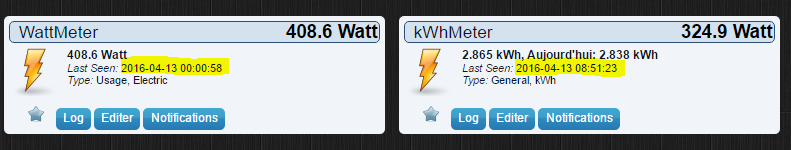• Hello chatainsim, in my domoticz i have only one device counting (kWh+instant Power)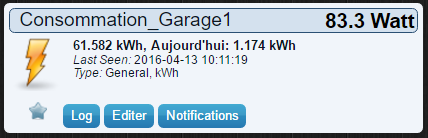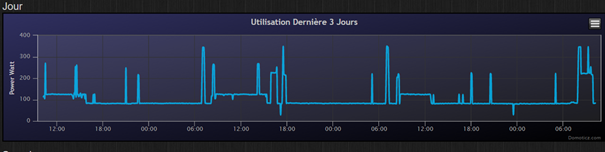The instant power updated every 90s is writing in Watt value(83.3) , the kWh of the day (1.174) and total energy (61.582 kWh).... the device log show the power = f(t).
Look if Watt value in (kWhMeter) device change, look the log graph ... if is ok, u not need Wattmeter device.
Thx Fredok

• @fredok Yes, the kWhMeter is working fine: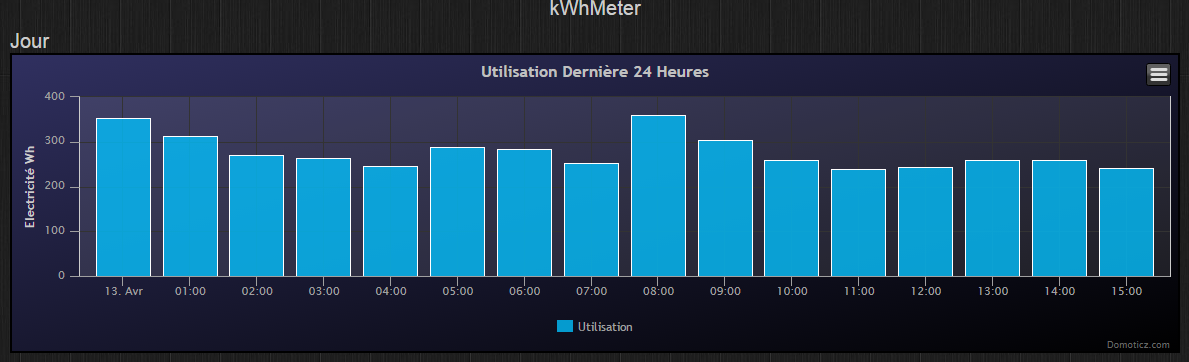• Ok, so it just stop working after almost 24 hours ...
I have some issue with mysensors, I don't know why, sometimes sensors stop working but I don't know why ...

``````send: 10-10-2-0 s=255,c=3,t=15,pt=2,l=2,sg=0,st=ok:0
send: 10-10-2-0 s=255,c=0,t=17,pt=0,l=5,sg=0,st=ok:1.5.4
send: 10-10-2-0 s=255,c=3,t=6,pt=1,l=1,sg=0,st=ok:2
sensor started, id=10, parent=2, distance=2
send: 10-10-2-0 s=255,c=3,t=11,pt=0,l=12,sg=0,st=ok:Energy Meter
send: 10-10-2-0 s=255,c=3,t=12,pt=0,l=3,sg=0,st=fail:1.0
send: 10-10-2-0 s=0,c=0,t=13,pt=0,l=0,sg=0,st=ok:
send: 10-10-2-0 s=0,c=2,t=24,pt=0,l=0,sg=0,st=fail:
send: 10-10-2-0 s=0,c=2,t=24,pt=0,l=0,sg=0,st=ok:
send: 10-10-2-0 s=0,c=2,t=24,pt=0,l=0,sg=0,st=ok:
``````

• hummm. i use this sketch with arduino mini near the GW (approx 5m max / 1 Wall) and i not see disconnection. So i cant help u.

• This is a recurring problem I have with MySensors.
All my sensors stop working, I have to clear the eprom then reupload the sketch.
Now It's working fine, until it fail again• Hello All,

I have tried this code and it works fine.
I was questionning myself though. If the index request fails at some point, what would be the behaviour of the code ? From my understanding of it, it will try to get the index again and again until it succeeds. May be a another way would be not to reset the index to 0 and send the additionnal index the next time.
What do you think ?

QQ.

• Hello, sorry for my late reply.

1. At the beginning, the sketch request the last kWh to the GW (I use Domoticz, so GW ask Domoticz the last kWh value) and send to the node.
2. If node receiv, the variable pcreceiv=true and it working fine else node wait for the request until he receiv. A serial.print will inform if receiv.

Fredok

• Yep, got that latelyThanks.

QQ.

• @fredok

hi , do you have please updated sketch for newest version of domoticz ? This old cause problems when I compiling it.

Thanks• @warmaniac
Sorry i not update this sketch ...

• @gohan

tried to modify but I always get error

/MySensors.h:328:2: error: #error No forward link or gateway feature activated. This means nowhere to send messages! Pretty pointless.

#error No forward link or gateway feature activated. This means nowhere to send messages! Pretty pointless.

``````// EmonLibrary examples openenergymonitor.org, Licence GNU GPL V3  ***/
#include <SPI.h>
#include <MySensors.h>
#include "EmonLib.h"             // Include Emon Library
EnergyMonitor emon1;             // Create an instance
#define CHILD_ID 0
#define PIN_ANALOG_I A1

//MySensor gw;
unsigned long lastSend;
unsigned long lastSend2;
unsigned long SEND_FREQUENCY = 20000; // Minimum time between send (in milliseconds). We don't wnat to spam the gateway.
unsigned long SEND_FREQUENCY2 = SEND_FREQUENCY / 25;
int index = 0;
double Irms=0;
double power;
boolean onyva=true;
float nrj=0, old_nrj;
MyMessage IrmsMsg(CHILD_ID,V_WATT);
MyMessage kWhMsg(CHILD_ID,V_KWH);
MyMessage pcMsg(CHILD_ID,V_VAR1);

//void incomingMessage(const MyMessage &message)

{
if (message.type==V_VAR1)
{
nrj = old_nrj = message.getFloat();
Serial.print("Received last nrj count from gw:");
Serial.println(nrj);
}
}

void presentation () {

sendSketchInfo("Energy Meter", "1.0");  // Send the sketch version information to the gateway and Controller
present(CHILD_ID, S_POWER);   // Register this device as power sensor
request(CHILD_ID, V_VAR1);

}

void setup()
{
begin(incomingMessage);
emon1.current(PIN_ANALOG_I, 30.0);       // Current: input pin, calibration.
}

void loop()
{
if (onyva) process();
onyva = false;
unsigned long now = millis();
//unsigned long now2 = millis();
bool sendTime2 = now - lastSend2 > SEND_FREQUENCY2;
if (sendTime2) //calcul Irms moy
{
if (index==0) Irms=emon1.calcIrms(1480);
else {
index++;
Irms = (index*Irms+emon1.calcIrms(1480))/(index+1);
}
lastSend2 = now;
}
bool sendTime = now - lastSend > SEND_FREQUENCY;
{
power = Irms*232.0;
send(IrmsMsg.set(power,1));
Serial.println(Irms*232.0);
nrj += (power*SEND_FREQUENCY/1000)/3.6E6;
send(kWhMsg.set(nrj,5));
send(pcMsg.set(nrj,5));
lastSend = now;
index = 0;
old_nrj=nrj;
onyva=true;
}
{
request(CHILD_ID, V_VAR1);
lastSend=now;
index=0;
onyva=true;
}
}
``````

It is possible to compile it with old mysensors library ? It will work with gateway which has a newest version ?

• Hello, at the end i write a v2.0 of the sketch

``````//#include <Arduino.h>
#define MY_DEBUG
// EmonLibrary examples openenergymonitor.org, Licence GNU GPL V3  ***/
#include <SPI.h>
#include <MySensors.h>
#include <DallasTemperature.h>
#include <OneWire.h>
#include "EmonLib.h"             // Include Emon Library
EnergyMonitor emon1;             // Create an instance
EnergyMonitor emon2;

#define CHILD_ID_CLAMP1 0
#define CHILD_ID_CLAMP2 1
#define CHILD_ID_TEMP 2
#define CHILD_ID_LDR 3

#define PIN_ANALOG_I1 A2 //pince amperemetrique1
#define PIN_ANALOG_I2 A3
#define PIN_LDR A0 // LDR
#define PIN_DALLAS 3

//Definition de dallas
#define MAX_ATTACHED_DS18B20 1
#define COMPARE_TEMP 1 // Send temperature only if changed? 1 = Yes 0 = No
OneWire oneWire(PIN_DALLAS);
DallasTemperature sensors(&oneWire);
float lastTemperature[MAX_ATTACHED_DS18B20];
int numSensors = 1;
bool metric = true; //truc v2.0

//int compat = 0;
unsigned long lastSend_temp = millis();
unsigned long lastSend_power = millis();
unsigned long lastSend_c;
unsigned long SEND_FREQUENCY = 120000; // Minimum time between send (in milliseconds). We don't wnat to spam the gateway.
unsigned long SEND_FREQUENCY_TEMP = 300000; //delais d'emission entre 2 mesures de temps
unsigned long SEND_FREQUENCY_C = SEND_FREQUENCY / 30;
int index = 0;
double Irms1 = 0;
double Irms2 = 0;
boolean first_time_temp = HIGH;

float nrj1 = 0, old_nrj1;
float nrj2 = 0, old_nrj2;
int ldr = 0, old_ldr;

MyMessage IrmsMsg1(CHILD_ID_CLAMP1, V_WATT);
MyMessage kWhMsg1(CHILD_ID_CLAMP1, V_KWH);
MyMessage pcMsg1(CHILD_ID_CLAMP1, V_VAR1);
MyMessage IrmsMsg2(CHILD_ID_CLAMP2, V_WATT);
MyMessage kWhMsg2(CHILD_ID_CLAMP2, V_KWH);
MyMessage pcMsg2(CHILD_ID_CLAMP2, V_VAR2);
MyMessage msgTemp(CHILD_ID_TEMP, V_TEMP);
MyMessage msgLight(CHILD_ID_LDR, V_LIGHT_LEVEL);

void before() {
sensors.begin();
}

void presentation()
{

sendSketchInfo("Multisensors_Entree", "2.0");  // Send the sketch version information to the gateway and Controller
present(CHILD_ID_CLAMP1, S_POWER);   // Register this device as power sensor
request(CHILD_ID_CLAMP1, V_VAR1);
present(CHILD_ID_CLAMP2, S_POWER);   // Register this device as power sensor
request(CHILD_ID_CLAMP2, V_VAR2);
numSensors = sensors.getDeviceCount(); //compte le nb de ds18
present(CHILD_ID_TEMP, S_TEMP);
present(CHILD_ID_LDR, S_LIGHT_LEVEL);
}

{
if (message.type == V_VAR1)
{
nrj1 = old_nrj1 = message.getFloat();
Serial.print("Received last nrj count from gw:");
Serial.println(nrj1);
}

if (message.type == V_VAR2)
{
nrj2 = old_nrj2 = message.getFloat();
Serial.print("Received last nrj count from gw:");
Serial.println(nrj2);
}

}

void setup()
{
sensors.setWaitForConversion(false);
emon1.current(PIN_ANALOG_I1, 30.0);       // Current: input pin, calibration.
emon2.current(PIN_ANALOG_I2, 30.0);
Serial.println("SETUP completed");
}

void envoi_donnees(double intrms, int id_clamp)
{
Serial.print("Envoi des donnees .... clamp="); Serial.println(id_clamp);
double energie = (intrms * 232.0 * SEND_FREQUENCY / 1000) / 3.6E6;
switch (id_clamp)
{
case 1:
{
send(IrmsMsg1.set(intrms * 232.0, 1));
nrj1 += energie;
send(kWhMsg1.set(nrj1, 5));
send(pcMsg1.set(nrj1, 5));
old_nrj1 = nrj1;
}
break;
case 2:
{
send(IrmsMsg2.set(intrms * 232.0, 1));
nrj2 += energie;
send(kWhMsg2.set(nrj2, 5));
send(pcMsg2.set(nrj2, 5));
old_nrj2 = nrj2;
}
break;
}
}

void loop() {
unsigned long now = millis();
bool sendTime_c = now - lastSend_c > SEND_FREQUENCY_C;
// Calcul de Irms1 et Irms2
if (sendTime_c) //calcul Irms moy clamp1/clamp2
{
if (index == 0)
{
Irms1 = emon1.calcIrms(1480);
Irms2 = emon2.calcIrms(1480);
}
else
{
Irms1 = (index * Irms1 + emon1.calcIrms(1480)) / (index + 1);
Irms2 = (index * Irms2 + emon2.calcIrms(1480)) / (index + 1);
}
lastSend_c = now;
index++;
}

bool sendTime_temp = now - lastSend_temp > SEND_FREQUENCY_TEMP;
if (sendTime_temp || first_time_temp)
{
Serial.println("........ quoiqu'il arrive, on envoi la temp+ldr");
first_time_temp = LOW;
//Send Temp
// Envoi Température d'après https://github.com/mysensors/MySensorsArduinoExamples/blob/master/examples/DallasTemperatureSensor/DallasTemperatureSensor.ino
sensors.requestTemperatures();
sleep(750);
for (int i = 0; i < numSensors && i < MAX_ATTACHED_DS18B20; i++)
{
// float temperature = static_cast<float>(static_cast<int>((getConfig().isMetric ? sensors.getTempCByIndex(i) : sensors.getTempFByIndex(i)) * 10.)) / 10.;
float temperature = static_cast<float>(static_cast<int>((getControllerConfig().isMetric?sensors.getTempCByIndex(i):sensors.getTempFByIndex(i)) * 10.)) / 10.;
if (lastTemperature[i] != temperature && temperature != -127.00 && temperature != 85.00)
{

// Send in the new temperature
send(msgTemp.setSensor(i).set(temperature, 1));
Serial.println(".....................Envoi Temp");
// Save new temperatures for next compare
lastTemperature[i] = temperature;
}
}

//Send light LDR
//Envoi Light LDR
ldr = (1023 - analogRead(PIN_LDR)) / 10.23;
if (old_ldr != ldr)
{
send(msgLight.set(ldr));
old_ldr = ldr;
}
lastSend_temp=now;
}

bool sendTime_power = now - lastSend_power > SEND_FREQUENCY;

if (sendTime_power)
{

// Envoi ou request Puissance1
else     {
request(CHILD_ID_CLAMP1, V_VAR1);
Serial.println("Request VAR1");
}

// Envoi ou request Puissance2
else     {
request(CHILD_ID_CLAMP2, V_VAR2);
Serial.println("Request VAR2");
}
//on reinitialise les compteurs
lastSend_power = now;
index = 0;
}
}

``````

attention, i use in the same node a ds18b20 for temp and LRD for light measurment
so u need to delete part of sketch.
i just compiling/Verifying this with mysensors v2.1 (but not loaded in arduino pro) and no error
for emonlib => https://github.com/openenergymonitor/EmonLib

• .... u must to look the monitor for see "Envoi des données .... clamp=0" and after (a few minutes after) "Envoi des données .... clamp=1"
Look after ur domotic soft for see if your gw become something.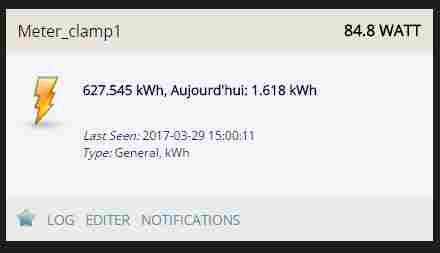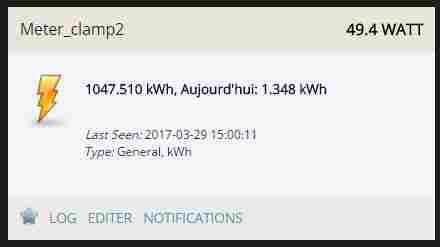bye.

• @fredok

nice ! thank you very much for fast solutionI try it at home , it compiles well• @warmaniac you got errors because you need to move the
"#include <MySensors.h>" after all the "#DEFINE....." otherwise the library does not know how to be configured

• @fredok

I modyfied your sketch (deleting unwanted code like dallas and light sensor) it works but i think I done something wrong, because it spams my gateway , now it looks like this

``````//#include <Arduino.h>
#define MY_DEBUG
// EmonLibrary examples openenergymonitor.org, Licence GNU GPL V3  ***/
#include <SPI.h>
#include <MySensors.h>
#include <OneWire.h>
#include "EmonLib.h"             // Include Emon Library
EnergyMonitor emon1;             // Create an instance

#define CHILD_ID_CLAMP1 0
#define PIN_ANALOG_I1 A1 //pince amperemetrique1

bool metric = true; //truc v2.0

//int compat = 0;
unsigned long lastSend_temp = millis();
unsigned long lastSend_power = millis();
unsigned long lastSend_c;
unsigned long SEND_FREQUENCY = 120000; // Minimum time between send (in milliseconds). We don't wnat to spam the gateway.
unsigned long SEND_FREQUENCY_C = SEND_FREQUENCY / 30;
int index = 0;
double Irms1 = 0;

float nrj1 = 0, old_nrj1;

MyMessage IrmsMsg1(CHILD_ID_CLAMP1, V_WATT);
MyMessage kWhMsg1(CHILD_ID_CLAMP1, V_KWH);
MyMessage pcMsg1(CHILD_ID_CLAMP1, V_VAR1);

void presentation()
{

sendSketchInfo("Multisensors_Entree", "2.0");  // Send the sketch version information to the gateway and Controller
present(CHILD_ID_CLAMP1, S_POWER);   // Register this device as power sensor
request(CHILD_ID_CLAMP1, V_VAR1);
}

{
if (message.type == V_VAR1)
{
nrj1 = old_nrj1 = message.getFloat();
Serial.print("Received last nrj count from gw:");
Serial.println(nrj1);
}

}

void setup()
{
emon1.current(PIN_ANALOG_I1, 30.0);       // Current: input pin, calibration.
Serial.println("SETUP completed");
}

void envoi_donnees(double intrms, int id_clamp)
{
Serial.print("Envoi des donnees .... clamp="); Serial.println(id_clamp);
double energie = (intrms * 232.0 * SEND_FREQUENCY / 1000) / 3.6E6;
switch (id_clamp)
{
case 1:
{
send(IrmsMsg1.set(intrms * 232.0, 1));
nrj1 += energie;
send(kWhMsg1.set(nrj1, 5));
send(pcMsg1.set(nrj1, 5));
old_nrj1 = nrj1;
}
break;
}
}

void loop() {
unsigned long now = millis();
bool sendTime_c = now - lastSend_c > SEND_FREQUENCY_C;
// Calcul de Irms1 et Irms2
if (sendTime_c) //calcul Irms moy clamp1/clamp2
{
if (index == 0)
{
Irms1 = emon1.calcIrms(1480);
}
else
{
Irms1 = (index * Irms1 + emon1.calcIrms(1480)) / (index + 1);
}
lastSend_c = now;
index++;
}

bool sendTime_power = now - lastSend_power > SEND_FREQUENCY;

if (sendTime_power)
{

// Envoi ou request Puissance1
else     {
request(CHILD_ID_CLAMP1, V_VAR1);
Serial.println("Request VAR1");
}

}
}
``````

and I get this results now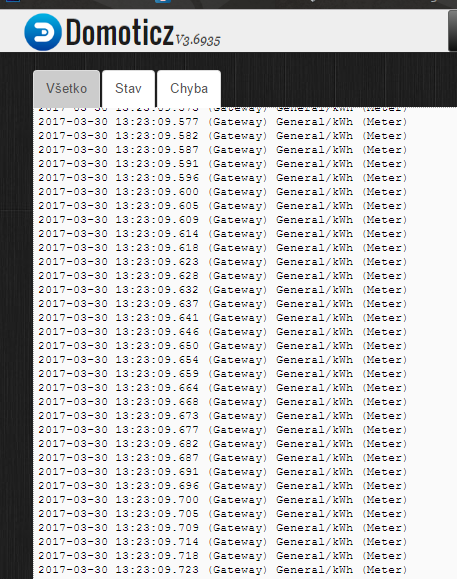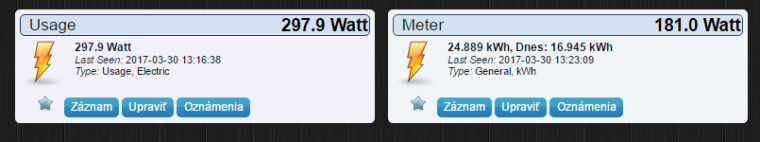• hello, i see the sketch send everyloop ???
try this

``````#define MY_DEBUG
// EmonLibrary examples openenergymonitor.org, Licence GNU GPL V3  ***/
#include <SPI.h>
#include <MySensors.h>
#include "EmonLib.h"             // Include Emon Library
EnergyMonitor emon1;             // Create an instance

#define CHILD_ID_CLAMP1 0
#define PIN_ANALOG_I1 A2 //pince amperemetrique1

unsigned long lastSend_power = millis();
unsigned long lastSend_c = millis();
unsigned long SEND_FREQUENCY = 120000; // Minimum time between send (in milliseconds). We don't wnat to spam the gateway.
unsigned long SEND_FREQUENCY_C = SEND_FREQUENCY / 30;
int index = 0;
double Irms1 = 0;
float nrj1 = 0, old_nrj1;

MyMessage IrmsMsg1(CHILD_ID_CLAMP1, V_WATT);
MyMessage kWhMsg1(CHILD_ID_CLAMP1, V_KWH);
MyMessage pcMsg1(CHILD_ID_CLAMP1, V_VAR1);

void before() {

}

void presentation()
{
sendSketchInfo("Multisensors_Entree", "2.0");  // Send the sketch version information to the gateway and Controller
present(CHILD_ID_CLAMP1, S_POWER);   // Register this device as power sensor
request(CHILD_ID_CLAMP1, V_VAR1);
}

{
if (message.type == V_VAR1)
{
nrj1 = old_nrj1 = message.getFloat();
Serial.print("Received last nrj count from gw:");
Serial.println(nrj1);
}
}

void setup()
{
emon1.current(PIN_ANALOG_I1, 30.0);       // Current: input pin, calibration.
Serial.println("SETUP completed");
}

void envoi_donnees(double intrms, int id_clamp)
{
Serial.print("Envoi des donnees .... clamp="); Serial.println(id_clamp);
double energie = (intrms * 232.0 * SEND_FREQUENCY / 1000) / 3.6E6;
switch (id_clamp) //can be modified ...
{
case 1:
{
send(IrmsMsg1.set(intrms * 232.0, 1));
nrj1 += energie;
send(kWhMsg1.set(nrj1, 5));
send(pcMsg1.set(nrj1, 5));
old_nrj1 = nrj1;
}
break;
}
}

void loop() {
unsigned long now = millis();
bool sendTime_c = now - lastSend_c > SEND_FREQUENCY_C;
// Calcul de Irms1 et Irms2
if (sendTime_c) //calcul Irms moy clamp1/clamp2
{
if (index == 0)
{
Irms1 = emon1.calcIrms(1480);
}
else
{
Irms1 = (index * Irms1 + emon1.calcIrms(1480)) / (index + 1);
}
lastSend_c = now;
index++;
}

bool sendTime_power = now - lastSend_power > SEND_FREQUENCY;

if (sendTime_power)
{

// Envoi ou request Puissance1
else     {
request(CHILD_ID_CLAMP1, V_VAR1);
Serial.println("Request VAR1");
}
//on reinitialise les compteurs
lastSend_power = now;
index = 0;
}
}
``````

if problem copy the screen of monitor.thx

• ahoj, if not working i will try with a new arduino for test the code.
dobrý večer

• @fredok

sketch works better now , not spamming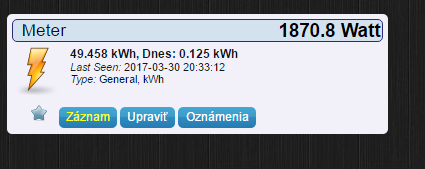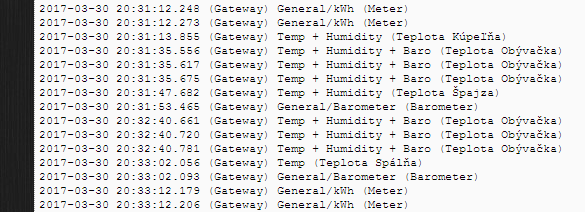but I dont understand current values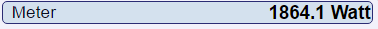• its seem good kWh every 2min
1864W is the power value

``````send(IrmsMsg1.set(intrms * 232.0, 1));
``````

the sketch send power value P (in W) and energy (in kWh) calculated over 2min.

``````double energie = (intrms * 232.0 * SEND_FREQUENCY / 1000) / 3.6E6;

// and after
nrj1 += energie;
send(kWhMsg1.set(nrj1, 5));
``````

in domoticz i have one "dispositif" included instant power Watt and day cumulate energy kWh
bye

• @fredok

Thank you very much! Only one question left, with multimeter I measured my voltage 241.0 V could I update it in sketch or ?

• a clamp measure only intensity I (in A) and Power =UrmsxIrms so if in u home Urms=241V u can change with this value but the power measurment not consider cosphy
by example the power measurment of my fridge =257W but true value is (with cosphy) 257x0,56=....W
so if u change 232V with 241V why not but at the end its will continu to be a approximate of the true power.
bye

• @fredok

Okayunderstand now, and what about calibration for wiring I followig this site

https://learn.openenergymonitor.org/electricity-monitoring/ct-sensors/how-to-build-an-arduino-energy-monitor-measuring-current-only?redirected=true

And they calibrate

`````` emon1.current(1, 111.1);
``````

And yours calibration

``````  emon1.current(PIN_ANALOG_I1, 30.0);
``````

Is some difference after this calibrations in measuring ?

• They also have the same sensor with also voltage measurement for a more accurate reading

• @gohan

Because with test sketch from that site I get actual consumption 0,5 kWh but from mysensors sketch I get much higher values look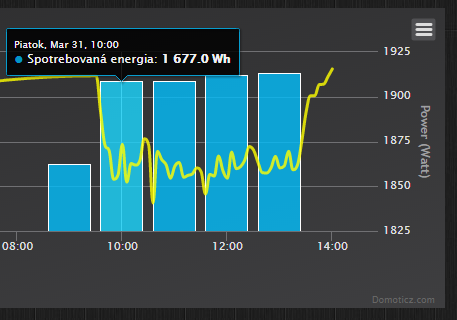1,7 kWh per one hour is too much , I had only TV and fridge in power on mode, I had total consumption per last year about 2140 kWh , which is about 6 kWh per day.

• hello,

1. the constant 30 is the divide between ratio/burden resistor including inside the clamp
for me ration=1800 and R=62ohm (1800/62 approx 30)
http://www.planete-domotique.com/notices/S/C/SCT013-030V.pdf
2. i can see 1677 Wh=1,677 kWh ... but 1900W for only TV+fridge its many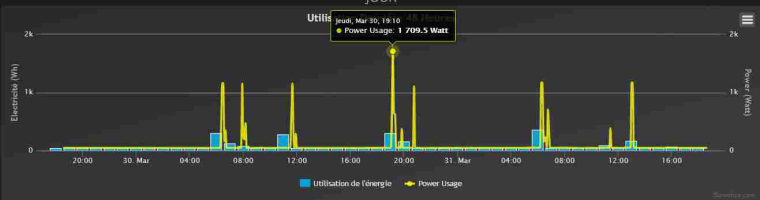3. Maybe it can help u??? i have a pulse powercounter count every flash (1 Wh of energy) of mine electric box and the power (Watt) send to gw is wrong in domoticz but the energy counting (kWh) is great.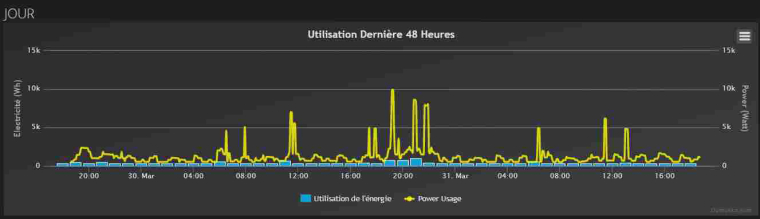Where come the wrong of fake power (GW ? sketch ? domoticz , i dont know)
bye

• @fredok

I had SCT-013-000 , not SCT-013-030 maybe this is the problem of measuring .

• updated my sketch with new values for SCT-013-00 result is now

``````#define MY_DEBUG
// EmonLibrary examples openenergymonitor.org, Licence GNU GPL V3  ***/
#include <SPI.h>
#include <MySensors.h>
#include "EmonLib.h"             // Include Emon Library
EnergyMonitor emon1;             // Create an instance

#define CHILD_ID_CLAMP1 0
#define PIN_ANALOG_I1 A1 //pince amperemetrique1

unsigned long lastSend_power = millis();
unsigned long lastSend_c = millis();
unsigned long SEND_FREQUENCY = 120000; // Minimum time between send (in milliseconds). We don't wnat to spam the gateway.
unsigned long SEND_FREQUENCY_C = SEND_FREQUENCY / 30;
int index = 0;
double Irms1 = 0;
float nrj1 = 0, old_nrj1;

MyMessage IrmsMsg1(CHILD_ID_CLAMP1, V_WATT);
MyMessage kWhMsg1(CHILD_ID_CLAMP1, V_KWH);
MyMessage pcMsg1(CHILD_ID_CLAMP1, V_VAR1);

void before() {

}

void presentation()
{
sendSketchInfo("Multisensors_Entree", "2.0");  // Send the sketch version information to the gateway and Controller
present(CHILD_ID_CLAMP1, S_POWER);   // Register this device as power sensor
request(CHILD_ID_CLAMP1, V_VAR1);
}

{
if (message.type == V_VAR1)
{
nrj1 = old_nrj1 = message.getFloat();
Serial.print("Received last nrj count from gw:");
Serial.println(nrj1);
}
}

void setup()
{
emon1.current(PIN_ANALOG_I1, 111.1);       // Current: input pin, calibration.
Serial.println("SETUP completed");
}

void envoi_donnees(double intrms, int id_clamp)
{
Serial.print("Envoi des donnees .... clamp="); Serial.println(id_clamp);
double energie = (intrms * 240.0 * SEND_FREQUENCY / 1000) / 3.6E6;
switch (id_clamp) //can be modified ...
{
case 1:
{
send(IrmsMsg1.set(intrms * 240.0, 1));
nrj1 += energie;
send(kWhMsg1.set(nrj1, 5));
send(pcMsg1.set(nrj1, 5));
old_nrj1 = nrj1;
}
break;
}
}

void loop() {
unsigned long now = millis();
bool sendTime_c = now - lastSend_c > SEND_FREQUENCY_C;
// Calcul de Irms1 et Irms2
if (sendTime_c) //calcul Irms moy clamp1/clamp2
{
if (index == 0)
{
Irms1 = emon1.calcIrms(1480);
}
else
{
Irms1 = (index * Irms1 + emon1.calcIrms(1480)) / (index + 1);
}
lastSend_c = now;
index++;
}

bool sendTime_power = now - lastSend_power > SEND_FREQUENCY;

if (sendTime_power)
{
// Envoi ou request Puissance1
else     {
request(CHILD_ID_CLAMP1, V_VAR1);
Serial.println("Request VAR1");
}
//on reinitialise les compteurs
lastSend_power = now;
index = 0;
}
}
``````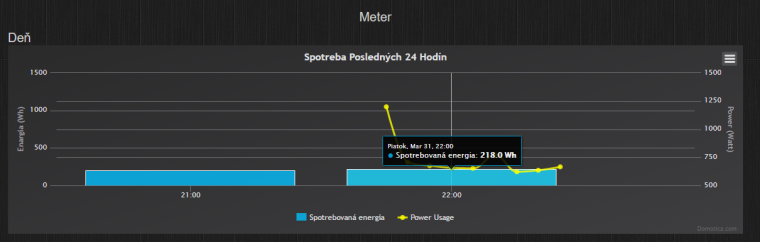• great !!!!!

• Hi , i update your sketch for Multiple sensor

``````#define MY_RADIO_NRF24
#define MY_DEBUG

#include <SPI.h>
#include <MySensors.h>
#include <EmonLib.h>

#define SERIAL_BAUD 115200

#define PIN_ANALOG_I1 A0   // The input you attached SCT1.
#define PIN_ANALOG_I2 A1   // The input you attached SCT2.
#define PIN_ANALOG_I3 A2   // The input you attached SCT3.

#define CHILD_ID_WATT 0    // child-id of sensor
#define CHILD_ID_KWH  1    // child-id of sensor
#define CHILD_ID_VAR1 2    // child-id of sensor

#define MAX_CT 3           // Number of CT sensor

EnergyMonitor ct1, ct2, ct3;

MyMessage msgWatt(CHILD_ID_WATT,V_WATT);
MyMessage msgKwh(CHILD_ID_KWH,V_KWH);
MyMessage msgVar(CHILD_ID_VAR1,V_VAR1);

unsigned long lastSend_power = millis();
unsigned long lastSend_c = millis();
unsigned long SEND_FREQUENCY   = 60000;               // 1 mn Minimum time between send (in milliseconds).
unsigned long FREQUENCY_SAMPLE = SEND_FREQUENCY / 12 ; // ( 5 s)

#define MAX_CT 3

double  Irms[MAX_CT];
float   nrj[MAX_CT];

int    index = 0;

void setup()
{
#ifdef MY_DEBUG
Serial.begin(SERIAL_BAUD);
Serial.println("Begin SETUP");
#endif
//ct1.current(PIN_ANALOG_I1, 111.1);     // Current: input pin, calibration.
ct1.current(PIN_ANALOG_I1, 60);          // current constant = (100 ÷ 0.050) ÷ 33 Homs = 60
ct2.current(PIN_ANALOG_I2, 60);
ct3.current(PIN_ANALOG_I3, 60);

Irms = ct1.calcIrms(1480);            // initial boot to charge up capacitor (no reading is taken) - testing
Irms = ct2.calcIrms(1480);
Irms = ct3.calcIrms(1480);

for (int i=0; i<MAX_CT; i++) {
Irms[i] = nrj[i] = 0;
}
#ifdef MY_DEBUG
Serial.println("SETUP completed");
#endif

}

void presentation() {

sendSketchInfo("Energy Meter", "2.1");

present(0, S_POWER);
request(0, V_VAR1);

present(1, S_POWER);
request(1, V_VAR1);

present(2, S_POWER);
request(2, V_VAR1);
}

int SensorID ;

SensorID = message.sensor ;

if (message.type == V_VAR1) {

nrj[SensorID] =  message.getFloat();

#ifdef MY_DEBUG
Serial.print(SensorID);
Serial.print("] count from GW:");
Serial.println(nrj[SensorID]);
#endif
}
}

void send_data(double intrms, int SensorID) {

#ifdef MY_DEBUG
Serial.print("Send data for SensorID=");
Serial.println(SensorID);
#endif

double energie = (intrms * 240.0 * SEND_FREQUENCY / 1000) / 3.6E6;

nrj[SensorID] += energie;
send(msgWatt.setSensor(SensorID).set(intrms * 240.0, 1));
send(msgKwh.setSensor(SensorID).set(nrj[SensorID], 5));
send(msgVar.setSensor(SensorID).set(nrj[SensorID], 5));
}

void loop() {

unsigned long now = millis();
double _Irms[MAX_CT];
bool sendTime_c = now - lastSend_c > FREQUENCY_SAMPLE;

if (sendTime_c)
{
_Irms = ct1.calcIrms(1480) ; if (_Irms < 0.3) _Irms = 0;
_Irms = ct2.calcIrms(1480) ; if (_Irms < 0.3) _Irms = 0;
_Irms = ct3.calcIrms(1480) ; if (_Irms < 0.3) _Irms = 0;

Irms = (index * Irms + _Irms) / (index + 1);
Irms = (index * Irms + _Irms) / (index + 1);
Irms = (index * Irms + _Irms) / (index + 1);

lastSend_c = now;
index++;

#ifdef MY_DEBUG
for (int i=0; i<MAX_CT; i++) {
Serial.print("CT");
Serial.print(i);  Serial.print(" : ");
Serial.print(Irms[i]*240.0);  // Apparent power
Serial.print(" ");
Serial.println(Irms[i]);      // Irms
}
#endif
}

bool sendTime_power = now - lastSend_power > SEND_FREQUENCY;

if (sendTime_power)
{
for (int i=0; i<MAX_CT; i++) {
// Envoi ou request Puissance1
{
send_data(Irms[i], i);
} else {
request(i, V_VAR1);
Serial.println("Request VAR1");
}
}
//on reinitialise les compteurs
lastSend_power = now;
index = 0;
}
}
``````

• fine thx....

9

1

1

5

21

6

9

5